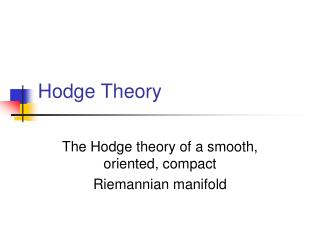Download PresentationHodge Theory

# Hodge Theory - PowerPoint PPT PresentationDownload Presentation## Hodge Theory

- - - - - - - - - - - - - - - - - - - - - - - - - - - E N D - - - - - - - - - - - - - - - - - - - - - - - - - - -
##### Presentation Transcript

1. Hodge Theory The Hodge theory of a smooth, oriented, compact Riemannian manifold

2. by William M. Faucette Adapted from lectures by Mark Andrea A. Cataldo

3. Structure of Lecture • The Inner Product on compactly supported forms • The adjoint dF • The Laplacian • Harmonic Forms • Hodge Orthogonal Decomposition Theorem • Hodge Isomorphism Decomposition Theorem • Poincaré Duality

4. The adjoint of d: d Let (M,g) be an oriented Riemannian manifold of dimension m. Then the Riemannian metric g on M defines a smoothly varying inner product on the exterior algebra bundle (TM*).

5. The adjoint of d: d The orientation on M gives rise to the F operator on the differential forms on M: In fact, the star operator is defined point-wise, using the metric and the orientation, on the exterior algebras (TM,q*) and it extends to differential forms.

6. The adjoint of d: d Note that in the example M=R with the standard orientation and the Euclidean metric shows that  and d do not commute. In particular, does not preserve closed forms.

7. The adjoint of d: d Define an inner product on the space of compactly supported p-forms on M by setting

8. The adjoint of d: d Definition: Let T:Ep(M)Ep(M) be a linear map. We say that a linear map is the formal adjoint to T with respect to the metric if, for every compactly supported u2Ep(M) and v2Ep(M)

9. The adjoint of d: d Definition: Define dF:Ep(M) Ep-1(M) by This operator, so defined, is the formal adjoint of exterior differentiation on the algebra of differential forms.

10. The adjoint of d: d Definition: The Laplace-Beltrami operator, or Laplacian, is defined as :Ep(M) Ep(M) by

11. The adjoint of d: d While F is defined point-wise using the metric, dF and  are defined locally (using d) and depend on the metric.

12. The adjoint of d: d Remark: Note that F= F. In particular, a form u is harmonic if and only if Fu is harmonic.

13. Harmonic forms and the Hodge Isomorphism Theorem

14. Harmonic forms and the Hodge Isomorphism Theorem Let (M,g) be a compact oriented Riemannian manifold. Definition: Define the space of real harmonic p-forms as

15. Harmonic forms and the Hodge Isomorphism Theorem Lemma: A p-form u2Ep(M) is harmonic if and only if du=0 and dFu=0. This follows immediately from the fact that

16. Harmonic forms and the Hodge Isomorphism Theorem Theorem: (The Hodge Orthogonal Decomposition Theorem) Let (M, g) be a compact oriented Riemannian manifold. Then and . . .

17. Harmonic forms and the Hodge Isomorphism Theorem we have a direct sum decomposition into  , -orthogonal subspaces

18. Harmonic forms and the Hodge Isomorphism Theorem Corollary: (The Hodge Isomorphism Theorem) Let (M, g) be a compact oriented Riemannian manifold. There is an isomorphism depending only on the metric g: In particular, dimRHp(M, R)<.

19. Harmonic forms and the Hodge Isomorphism Theorem Theorem: (Poincaré Duality) Let M be a compact oriented smooth manifold. The pairing is non-degenerate.

20. Harmonic forms and the Hodge Isomorphism Theorem In fact, the F operator induces isomorphisms for any compact, smooth, oriented Riemannian manifold M. The result follows by the Hodge Isomorphism Theorem.